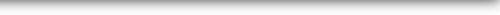DASP

Distributive Analysis Stata PackageDASP Modules

## Inequality

IATKINSON Estimates the Atkinson indices and their standard errors taking full account of survey design.

DIATKINSON Estimates the difference between Atkinson indices and its standard error taking full account of survey design.

## Polarisation

IPOLFW Estimates the Foster and Wolfson - FW- (1992) index and its standard errors taking full account of survey design.

DIPOLFW Estimates the difference between FW indices and its standard error taking full account of survey design.

IPOEGR Estimates the Esteban, Gardin and Ray - EGR- (1999) index and its standard errors taking full account of survey design.

## Poverty elasticities

EFGTGR

FGT-Elasticity with respect to growth in average income (Kakwani (1993)).

EFGTINEQ

FGT-Elasticity with respect to Gini inequality (Kakwani (1993)).

## Poverty targeting

ITARGETG

The module itargetg produces the following estimates and curves for a given list of population groups:

• Impact of targeting population groups on FGT indice

• Impact of targeting population groups on FGT curves (along an axis of poverty lines)

• The confridence interval of the impact of targeting population groups on FGT curves (along an axis of poverty lines).

ITARGETC

The module itargetc produces the following estimates and curves for a given list of population groups:

• Impact of targeting welfare components on FGT indice;

• Impact of targeting welfare components on on FGT curves (along an axis of poverty lines);

• The confidence interval of targeting welfare components on FGT curves (along an axis of poverty lines).

## Decomposition

DFGTS

The command dfgts decomposes the allevation of FGT poverty by income components and provides standard errors on elements of the decompositions. Without any source, the FGT index equals 1. The decomposition is performed using the Shapley value.

DSGINIS

Decomposes the Gini index by income sources with the Shapley approach.

DPOLAG Decomposes the Duclos, Esteban amd Ray polarization index  by population subgroups (Araar (2008) aproach).

DPOLAS

Decomposes the Duclos, Esteban amd Ray polarization index  by income components (Araar (2008) aproach).

## Curves

CLORENZSM

Produces the following curves with confidence interval

• Lorenz curves;

• Generalized Lorenz curves;

• Concentration curves;

• Generalized concentration curves.

CFGTSM

Produces the following curves with confidence interval

• Normalised FGT curves;

• FGT curves.

CDOMC

Produces the following curve with confidence interval

• Normalised consumption dominance curves;

• Consumption dominance curves.

CDOMC2D

Produces the difference between the following curve with confidence interval

• Normalised consumption dominance curves;

• Consumption dominance.

## Distributive tools

QUINSH

Estimate the income shares and cumulative income shares by group quantiles (quartiles/quintiles/deciles/etc.). Graph bar of income shares can be plotted.

## Disaggregated data

UNGROUP

The command ungroup generates disaggregated data using information on the aggregated data and on the form of the distribution to be assumed.

Options
• The user can perform the statistical tests  for the difference between indices, and this, by adding the option: test(value). For instance, the user may be interested to test whether the variation in Gini between two periods is higher, equals or lower than 0.01;

• For the plotted curves by groups, the correspondent curve at population level is added.